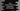# Java stream findFirst() explanation with example## Java stream findFirst() explanation with example:

findFirst() is used to find the first element in a stream in Java. It returns one Optional value holding the element found. If the stream is empty, it will return one empty optional. In this post, I will show you how findFirst() works with an example.

### Definition of findFirst:

findFirst is defined as below:

``Optional<T> findFirst()``
• It returns an optional value holding the element from the stream.
• It returns one empty optional if the stream is empty.
• It returns NullPointerException if the selected element is null.

### Example of findFirst:

Let’s consider the below example program:

``````package com.company;

import java.util.Arrays;
import java.util.Optional;
import java.util.stream.Stream;

public class Main {
public static void main(String[] args){
Stream<Integer> intStream = Arrays.asList(10, 20, 30, 40, 50, 40).stream();

Optional firstValue = intStream.findFirst();

System.out.println(firstValue);
}
}``````

It will print Optional(10).

We can also check if a value exists or not using isPresent() and get the value using get():

``````package com.company;

import java.util.Arrays;
import java.util.Optional;
import java.util.stream.Stream;

public class Main {
public static void main(String[] args){
Stream<Integer> intStream = Arrays.asList(10, 20, 30, 40, 50, 40).stream();

Optional firstValue = intStream.findFirst();

if(firstValue.isPresent()){
System.out.println(firstValue.get());
}
}
}``````

It will print 10.

### Example of using filter with findFirst:

We can also use filter with findFirst. filter can filter out a value in the stream and if we use findFirst with filter, we can get the first value that matches the filter condition.

For example:

``````package com.company;

import java.util.Arrays;
import java.util.Optional;
import java.util.stream.Stream;

public class Main {
public static void main(String[] args){
Stream<Integer> intStream = Arrays.asList(1, 4, 6, 7, 3, 9, 10).stream();

Optional firstValue = intStream.filter(x -> x%2 == 0).findFirst();

if(firstValue.isPresent()){
System.out.println(firstValue.get());
}
}
}``````

Here, we are using filter and findFirst to find the first even number in the number list. It will print 4 as the output.